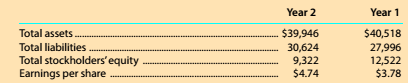Chapter 8, Problem 8.8.2MBA

Chapter
Section
Textbook Problem

Debt and price-earnings ratios The Home Depot. Inc. (HD) operates over 2.200 home improvement retail stores and is a competitor of Lowe's (LOW). The following data (in millions) were adapted from recent financial statements of The Home Depot.Given your answer lo part (1), what is the ratio of stockholders' equity to total assets? Round to one decimal place.

To determine

Concept Introduction:

Debt Ratio:

Debt ratio is the relationship between the Total liabilities and Total Assets of a corporation. Debt ratio shows the part of assets financed by debts. It is calculated by dividing total liabilities by total assets. The formula of debt ratio is as follows:

Debt Ratio = Total LiabilitiesTotal Assets

To Calculate:

The Stockholder's Equity to total asset ratio using the Debt Ratios for year 1 and 2

Explanation

The Stockholder's Equity to total asset ratio using the Debt Ratios for year 1 and 2 is calculated as follows;

 $in Millions Year 1 Year 2 Total Liabilities (A)$30,624 \$27,996

Still sussing out bartleby?

Check out a sample textbook solution.

See a sample solution

The Solution to Your Study Problems

Bartleby provides explanations to thousands of textbook problems written by our experts, many with advanced degrees!

Get Started

Find more solutions based on key concepts